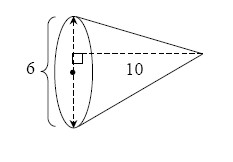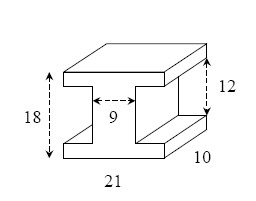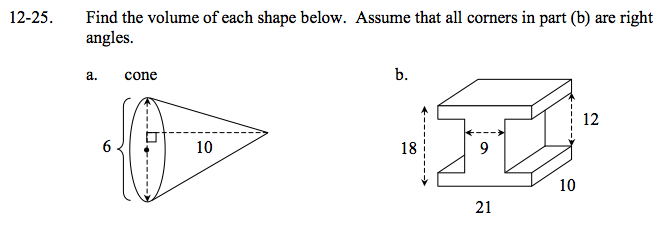### Home > GC > Chapter 12 > Lesson 12.1.3 > Problem12-25

12-25.
1. Find the volume of each shape below. Assume that all corners in part (b) are right angles. Homework Help ✎

1. cone2.$V= \frac{ 1 }{3 }(\pi)(3^2)(10) = 30 \pi = 94.2 \text{ un}^3$

What is the formula for the volume of a cone?

Find the volume of a 18 by 21 by 10 rectangular prism, then subtract the missing parts.

2340 un3